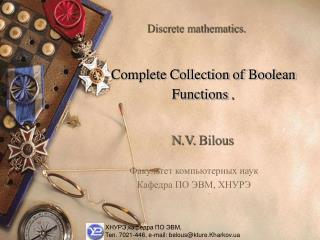DownloadDownload PresentationХНУРЭ,кафедра ПО ЭВМ, Тел . 7021-446, e-mail: belous@kture.Kharkov.ua

# ХНУРЭ,кафедра ПО ЭВМ, Тел . 7021-446, e-mail: belous@kture.Kharkov.ua

Télécharger la présentation## ХНУРЭ,кафедра ПО ЭВМ, Тел . 7021-446, e-mail: belous@kture.Kharkov.ua

- - - - - - - - - - - - - - - - - - - - - - - - - - - E N D - - - - - - - - - - - - - - - - - - - - - - - - - - -
##### Presentation Transcript

1. Discrete mathematics. Complete Collection of Boolean Functions. N.V. Bilous Факультеткомпьютерных наук Кафедра ПО ЭВМ, ХНУРЭ ХНУРЭ,кафедра ПО ЭВМ, Тел. 7021-446, e-mail: belous@kture.Kharkov.ua

2. The conception of completeness collection of Boolean function. A collection of Boolean functions is called complete if an arbitrary Boolean function can be represented as a superposition of the functions from this collection.

3. The conception of completeness collection of Boolean function. Example. Prove that the collections {,¯}, {,¯} – are complete if it is known that the collection {,,¯} is complete.

4. The conception of completenesscollection of Boolean function. Solution. Represent functionby the means of the collection {, ¯} according to De Morgan’s law: Hence, all functions of the complete collection{,,¯}may be represented with the help of the collection {, ¯}.

5. The conception of completenesscollection of Boolean function. Continuation of example. By analogy completeness of the collection {, ¯} is proved, sofunctionmay be representedby the collection {, ¯} with the help of De Morgan’s law in the following form :

6. The conception of closed class of Boolean functions. Closure of set  Boolean functions is called a set [] consisting of functions which may be obtained by the means of superposition of function from . A set  of Boolean functions is called a closed class if any superposition of the functions from the set  is a function, that also belongs to .

7. The conception of closed class of Boolean functions. There are five main closed classes of Boolean functions: 1. Selfdual functions. 2. Linear functions. 3. Functions, that retain zero. 4. Functions, that retain unity. 5. Monotonic functions.

8. Monotonic Boolean functions. Boolean functionfis calledmonotonic if for any pairs of Boolean constants (a1,…,an) and (b1,…,bn) an order relation is taken place (a1,…,an)  (b1,…,bn), and the inequality f(a1,…,an) f(b1,…,bn) is right.

9. Monotonic Boolean functions. Example. Verify if the functions f(x,y)=xy, g(x,y)= xy are monotonic or not. Solution. (0,0)(0,1),f(0,0)=0, f(0,1)=0,f(0,0)f (0,1). (0,0)(1,0),f(0,0)=0, f(1,0)=0,f(0,0)f (1,0). (0,0)(1,1), f(0,0)=0, f(1,1)=1,f(0,0)f (1,1). (0,1)(1,1),f(0,1)=0, f(1,1)=1,f(0,1)f(1,1). (1,0)(1,1),f(1,0)=0, f(1,1)=1,f(1,0)f(1,1). Conclusion: the function f(x,y)=xy is monotonic.

10. Monotonic Boolean functions. Continuation of example. By analogy verify the function g(x,y). (0,0)(0,1),g(0,0)=0 g (0,1)=1,g(0,0)g (0,1). (0,0)(1,0), g(0,0)=0 g (1,0)=1,g(0,0)g (1,0). (0,0)(1,1),g(0,0)=0 g (1,1)=0,g(0,0)g (1,1). (0,1)(1,1),g(0,1)=1 g(1,1)=0,g(0,1)g(1,1). Conclusion: functiong(x,y)=xy is not monotonic.

11. Boolean functions that retain zero and unity. Boolean functionf(x1,x2,...,xn) is called function that retain zero if f(0, 0,..., 0)=0. Boolean functionf(x1,x2,...,xn) is called function that retain unityif f(1, 1, ..., 1)=1.

12. Boolean functions that retain zero and unity. Example. Define, if the function retains 0 and 1. Solution. Hence, given function retains 1, and does not retain 0.

13. Post’s theorem about complete collection of Boolean function. According to the Post’s theorem, a collection of Boolean functions is complete if it contains a) at least one not linear function, b) at least one not selfdual function, c) at least one not monotonic function, d) at least one function that does not retain zero, e)at least one function that does not retain unity. Exampleof defining complete collection of Boolean functions